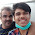JAVA Concepts (Worksheet-2)

Q1: See the following Java code. Identify
(i) Superclass
(ii) Subclass
(iii) Can student class modify the value of birthyear? Why or Why not?
(iv)    Can student class modyfy the value of age? Why or Why not?

class human

private int birthyear;
String name;
int age;
void method1()
{...}
}

class student extends human
{
int rollno;
...
void method2()
{...}
}

Q2: While defining a class, if an instance variable is to be used only by methods defined within its class, then what type access specifier be declared for that variable?

Q3: Write command to display a message dialog to display prompt as “Hello World” , title as “My dialog” and icon as question icon.

Q4: Write code to add an element (“New Course”) to a list (SubList) at the beginning of the list.

Q5: What is the difference between ‘a’ and “a” ?

Q6: Name the inheritance type which is not supported by JAVA.

Q7: What is an Event?

Q8: How GUI application works?

Q9: What do you mean by parsing? Give two examples at least.

Q10: What is an expression? What are the various types of expressions in Java?

Q11: Write a java program to calculate the sum of all the no. divisible by 5 in the range 1 to 50. Show result to JLabel type GUI element.

Q12: How many times, the following loop gets executed?
int i=0;
do

//Statements
} while(i>20);

Q13: What will be the value of j and k after execution of the following code:
intj=10,k=12;
if(k>=j)
{
k=j;
J=k;
}1 comment:

1.